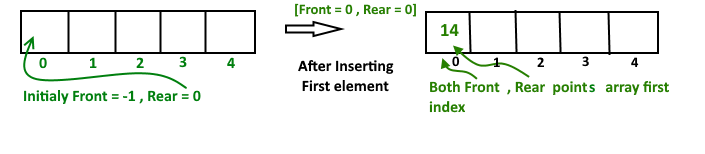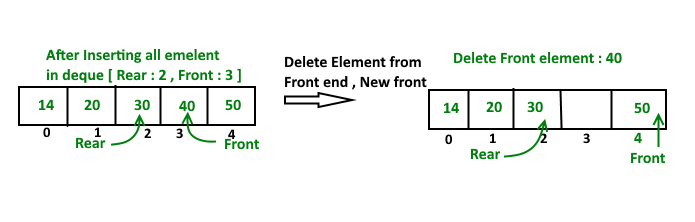Deque | Introduction and Applications

Deque or Double Ended Queue is a generalized version of the Queue data structure that allows insertion and deletion at both the ends.

Operations on Deque: Mainly the following four basic operations are performed on deque.

insetFront(): Adds an item at the front of the Deque.
insertLast(): Adds an item at the rear of the Deque.
deleteFront(): Deletes an item from front of the Deque.
deleteLast(): Deletes an item from rear of the Deque.

In addition to above operations, following operations are also supported by deque.
getFront(): Gets the front item/data from the queue.
getRear(): Gets the last item/data from the queue.
isEmpty(): Checks whether the Deque is empty or not.
isFull(): Checks whether the Deque is full or not.

Applications of Deque:

Since Deque supports both stack and queue operations, it can be used as both. The Deque data structure supports clockwise and anticlockwise rotations in O(1) time which can be useful in certain applications. Also, the problems where elements need to be removed and or added from both the ends can be efficiently solved using Deque.

Implementation:

A Deque can be implemented either using a doubly linked list or circular array. In both implementation, we can implement all operations in O(1) time. C/C++ implementation of Deque Data structure is given below

Implementation of Deque using circular array

To implement a deque, we need to keep track of two indices, front and rear. We enqueue (push) an item at the rear or the front end of deque and dequeue(pop) an item from both rear and front end.

Steps Involved:

1. Create an empty array ‘arr’ of size ‘n’
initialize front = -1 , rear = 0
Inserting First element in deque either front end or read end they both lead to the same result.After insert Front Points = 0 and Rear points = 0

Insert Elements at Rear end

a). First we check deque if Full or Not
b). IF Rear == Size-1
then reinitialize Rear = 0 ;
Else increment Rear by '1'
and push current key into Arr[ rear ] = key
Front remain same.

Insert Elements at Front end

a). First we check deque if Full or Not
b). IF Front == 0 || initial position, move Front
to points last index of array
front = size - 1
Else decremented front by '1' and push
current key into Arr[ Front] = key
Rear remain same.C ++

 // C++ implementation of De-queue using circular// array#includeusing namespace std; // Maximum size of array or Dequeue#define MAX 100 // A structure to represent a Dequeclass Deque{    int  arr[MAX];    int  front;    int  rear;    int  size;public :    Deque(int size)    {        front = -1;        rear = 0;        this->size = size;    }     // Operations on Deque:    void  insertfront(int key);    void  insertrear(int key);    void  deletefront();    void  deleterear();    bool  isFull();    bool  isEmpty();    int  getFront();    int  getRear();}; // Checks whether Deque is full or not.bool Deque::isFull(){    return ((front == 0 && rear == size-1)||            front == rear+1);} // Checks whether Deque is empty or not.bool Deque::isEmpty (){    return (front == -1);} // Inserts an element at frontvoid Deque::insertfront(int key){    // check whether Deque if  full or not    if (isFull())    {        cout << "Overflow\n" << endl;        return;    }     // If queue is initially empty    if (front == -1)    {        front = 0;        rear = 0;    }     // front is at first position of queue    else if (front == 0)        front = size - 1 ;     else // decrement front end by '1'        front = front-1;     // insert current element into Deque    arr[front] = key ;} // function to inset element at rear end// of Deque.void Deque ::insertrear(int key){    if (isFull())    {        cout << " Overflow\n " << endl;        return;    }     // If queue is initially empty    if (front == -1)    {        front = 0;        rear = 0;    }     // rear is at last position of queue    else if (rear == size-1)        rear = 0;     // increment rear end by '1'    else        rear = rear+1;     // insert current element into Deque    arr[rear] = key ;} // Deletes element at front end of Dequevoid Deque ::deletefront(){    // check whether Deque is empty or not    if (isEmpty())    {        cout << "Queue Underflow\n" << endl;        return ;    }     // Deque has only one element    if (front == rear)    {        front = -1;        rear = -1;    }    else        // back to initial position        if (front == size -1)            front = 0;         else // increment front by '1' to remove current            // front value from Deque            front = front+1;} // Delete element at rear end of Dequevoid Deque::deleterear(){    if (isEmpty())    {        cout << " Underflow\n" << endl ;        return ;    }     // Deque has only one element    if (front == rear)    {        front = -1;        rear = -1;    }    else if (rear == 0)        rear = size-1;    else        rear = rear-1;} // Returns front element of Dequeint Deque::getFront(){    // check whether Deque is empty or not    if (isEmpty())    {        cout << " Underflow\n" << endl;        return -1 ;    }    return arr[front];} // function return rear element of Dequeint Deque::getRear(){    // check whether Deque is empty or not    if(isEmpty() || rear < 0)    {        cout << " Underflow\n" << endl;        return -1 ;    }    return arr[rear];} // Driver program to test above functionint main(){    Deque dq(5);    cout << "Insert element at rear end  : 5 \n";    dq.insertrear(5);     cout << "insert element at rear end : 10 \n";    dq.insertrear(10);     cout << "get rear element " << " "         << dq.getRear() << endl;     dq.deleterear();    cout << "After delete rear element new rear"         << " become " << dq.getRear() << endl;     cout << "inserting element at front end \n";    dq.insertfront(15);     cout << "get front element " << " "         << dq.getFront() << endl;     dq.deletefront();     cout << "After delete front element new "       << "front become " << dq.getFront() << endl;    return 0;}

Java

 // Java implementation of De-queue using circular// array  // A structure to represent a Dequeclass Deque{    static final int MAX = 100;    int  arr[];    int  front;    int  rear;    int  size;         public Deque(int size)    {        arr = new int[MAX];        front = -1;        rear = 0;        this.size = size;    }      /*// Operations on Deque:    void  insertfront(int key);    void  insertrear(int key);    void  deletefront();    void  deleterear();    bool  isFull();    bool  isEmpty();    int  getFront();    int  getRear();*/      // Checks whether Deque is full or not.    boolean isFull()    {        return ((front == 0 && rear == size-1)||            front == rear+1);    }      // Checks whether Deque is empty or not.    boolean isEmpty ()    {        return (front == -1);    }      // Inserts an element at front    void insertfront(int key)    {        // check whether Deque if  full or not        if (isFull())        {            System.out.println("Overflow");             return;        }          // If queue is initially empty        if (front == -1)        {            front = 0;            rear = 0;        }                 // front is at first position of queue        else if (front == 0)            front = size - 1 ;          else // decrement front end by '1'            front = front-1;          // insert current element into Deque        arr[front] = key ;    }      // function to inset element at rear end    // of Deque.    void insertrear(int key)    {        if (isFull())        {            System.out.println(" Overflow ");            return;        }          // If queue is initially empty        if (front == -1)        {            front = 0;            rear = 0;        }          // rear is at last position of queue        else if (rear == size-1)            rear = 0;          // increment rear end by '1'        else            rear = rear+1;                 // insert current element into Deque        arr[rear] = key ;    }      // Deletes element at front end of Deque    void deletefront()    {        // check whether Deque is empty or not        if (isEmpty())        {            System.out.println("Queue Underflow\n");            return ;        }          // Deque has only one element        if (front == rear)        {            front = -1;            rear = -1;        }        else            // back to initial position            if (front == size -1)                front = 0;              else // increment front by '1' to remove current                // front value from Deque                front = front+1;    }      // Delete element at rear end of Deque    void deleterear()    {        if (isEmpty())        {            System.out.println(" Underflow");            return ;        }          // Deque has only one element        if (front == rear)        {            front = -1;            rear = -1;        }        else if (rear == 0)            rear = size-1;        else            rear = rear-1;    }      // Returns front element of Deque    int getFront()    {        // check whether Deque is empty or not        if (isEmpty())        {            System.out.println(" Underflow");            return -1 ;        }        return arr[front];    }      // function return rear element of Deque    int getRear()    {        // check whether Deque is empty or not        if(isEmpty() || rear < 0)        {            System.out.println(" Underflow\n");            return -1 ;        }        return arr[rear];    }      // Driver program to test above function    public static void main(String[] args)    {                  Deque dq = new Deque(5);                   System.out.println("Insert element at rear end  : 5 ");         dq.insertrear(5);                   System.out.println("insert element at rear end : 10 ");         dq.insertrear(10);                   System.out.println("get rear element : "+ dq.getRear());                   dq.deleterear();         System.out.println("After delete rear element new rear become : " +                                 dq.getRear());                   System.out.println("inserting element at front end");         dq.insertfront(15);                   System.out.println("get front element: " +dq.getFront());                   dq.deletefront();                   System.out.println("After delete front element new front become : " +                                    +  dq.getFront());             }}

Output:

insert element at rear end  : 5
insert element at rear end : 10
get rear element : 10
After delete rear element new rear become : 5
inserting element at front end
get front element : 15
After delete front element new front become : 5

Relevant exercises

POST A NEW COMMENT

• Input (stdin)

Output (stdout)

Input (stdin)

Expected Output

Compiler Message

Input (stdin)

2    3